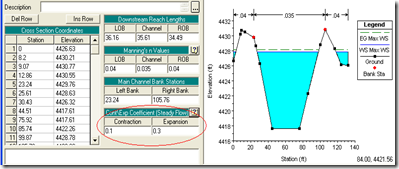## Thursday, June 24, 2010

### Contraction and Expansion Losses for Unsteady Flow

Written by Chris Goodell, P.E., D. WRE | WEST Consultants

Since unsteady flow was introduced in HEC-RAS years ago, the contraction and expansion loss coefficients were not used, because losses due to contraction and expansion were automatically approximated in the conservation of momentum equation. Since steady flow RAS does not use the momentum equation for backwater computations, we had to approximate the contraction and expansion losses using those loss coefficients that you see for every cross section in the cross section editor. When switching to unsteady flow, you could leave those coefficients in every cross section; RAS just won’t use them.In the latest version of RAS (version 4.1), the release notes indicate that RAS may not be capturing all of the C&E losses in unsteady flow, particularly at sharp contractions and expansion. And therefore, unsteady contraction and expansion loss coefficients can now be used.

From the 4.1 Release notes:, “In general, contraction and expansion losses are not used in unsteady flow, and therefore the default coefficients are 0.0. Forces due to contractions and expansions are handled in the momentum equation through pressure force differences. However, because HEC-RAS is a one-dimensional unsteady flow model, the one-dimensional momentum equation does not always capture all of the forces action on the flow field at a sharp contraction and/or expansion zone. In order to better approximate the forces acting on the water, and the resulting water surface elevation, at a contraction and/or expansion, the user can enter empirical contraction and expansion coefficients for unsteady flow modeling. These coefficients will be multiplied by a change in velocity head, just like in steady flow modeling, but the resulting energy loss gets converted to an equivalent force for placement into the momentum equation.”

Notice that there is a new table for entering unsteady flow contraction and expansion losses.So the obvious question is, “what values do we use for unsteady flow contraction and expansion coefficients?” Are they the same as their steady flow counterparts? Also, when do we want to use them?

Any suggestions out there???